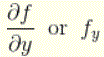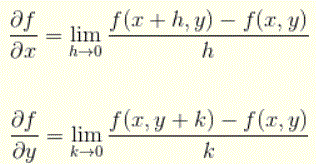# Partial Derivatives

## Definition of Partial Derivatives

Let f(x,y) be a function with two variables. If we keep y constant and differentiate f (assuming f is differentiable) with respect to the variable x, using the rules and formulas of differentiation, we obtain what is called the partial derivative of f with respect to x which is denoted bySimilarly If we keep x constant and differentiate f (assuming f is differentiable) with respect to the variable y, we obtain what is called the partial derivative of f with respect to y which is denoted byWe might also use the limits to define partial derivatives of function f as follows:## Examples with Detailed Solutions

We now present several examples with detailed solution on how to calculate partial derivatives.

### Example 1

Find the partial derivatives f x and f y if f(x , y) is given by
f(x,y) = x^2 y + 2 x + y

Solution to Example 1:
Assume y is constant and differentiate with respect to x to obtain
f_x = \frac{\partial f}{\partial x} = \frac{\partial}{\partial x}(x^2 y + 2 x + y ) \\\\ = \frac{\partial}{\partial x}(x^2 y ) + \frac{\partial}{\partial x}(2 x) + \frac{\partial}{\partial x}( y ) = 2 xy + 2 + 0 = 2xy + 2
Now assume x is constant and differentiate with respect to y to obtain
f_y = \frac{\partial f}{\partial y} = \frac{\partial}{\partial y}(x^2 y + 2 x + y ) \\\\ = \frac{\partial}{\partial y}(x^2 y ) + \frac{\partial}{\partial y}(2 x) + \frac{\partial}{\partial y}( y ) = x^2 + 0 + 1 = x^2 + 1

### Example 2

Find f x and f y if f(x , y) is given by
f(x,y) = \sin(x y) + \cos x

Solution to Example 2:
Differentiate with respect to x assuming y is constant
f_x = \frac{\partial f}{\partial x} = \frac{\partial}{\partial x}(\sin(x y) + \cos x ) \\\\ = \frac{\partial}{\partial x}(\sin(x y) ) + \frac{\partial}{\partial x}(\cos x) = y \cos(x y) -\sin(x)

Differentiate with respect to y assuming x is constant
f_y = \frac{\partial f}{\partial y} = \frac{\partial}{\partial y}(\sin(x y) + \cos x ) \\\\ = \frac{\partial}{\partial y}(\sin(x y) ) + \frac{\partial}{\partial y}(\cos x) = x \cos(x y) - 0 = x \cos(x y)

### Example 3

Find f x and f y if f(x , y) is given by
f(x,y) = x e^{x y}

Solution to Example 3:
Differentiate with respect to x assuming y is constant using the product rule of differentiation.

f_x = \frac{\partial f}{\partial x} = \frac{\partial}{\partial x}(x e^{x y}) \\\\ = \frac{\partial}{\partial x}(x) e^{x y} + x \frac{\partial}{\partial x}(e^{x y}) = 1 \cdot e^{x y} + x \cdot y e^{x y} = (1+xy) e^{x y}

Differentiate with respect to y assuming x is constant.
f_y = \frac{\partial f}{\partial y} = \frac{\partial}{\partial y}(x e^{x y}) \\\\ = x \frac{\partial}{\partial y}(e^{x y}) = x \cdot x e^{x y} = x^2 e^{x y}

### Example 4

Find f x and f y if f(x , y) is given by
f(x,y) = \ln(x^2+2y)

Solution to Example 4:
Differentiate with respect to x to obtain

f_x = \frac{\partial f}{\partial x} = \frac{\partial}{\partial x}(\ln(x^2+2y)) \\\\ = \frac{\partial}{\partial x}(x^2+2y) \cdot \dfrac{1}{x^2+2y} = \dfrac{2x}{x^2+2y}

Differentiate with respect to y
f_y = \frac{\partial f}{\partial y} = \frac{\partial}{\partial y}(\ln(x^2+2y)) \\\\ = \frac{\partial}{\partial y}(x^2+2y) \cdot \dfrac{1}{x^2+2y} = \dfrac{2}{x^2+2y}

### Example 5

Find f x (2 , 3) and f y (2 , 3) if f(x , y) is given by
f(x,y) = y x^2 + 2 y

Solution to Example 5:
We first find the partial derivatives fx and fy
f
x (x,y) = 2x y
f
y (x,y) = x 2 + 2
We now calculate fx(2 , 3) and fy(2 , 3) by substituting x and y by their given values
f
x (2,3) = 2 (2)(3) = 12
f
y (2,3) = 2 2 + 2 = 6

## Exercises

Find partial derivatives f x and f y of the following functions
1. f(x , y) = x e
x + y
2. f(x , y) = ln ( 2 x + y x)
3. f(x , y) = x sin(x - y)

### Answers to the Above Exercises

1. f x =(x + 1)e x + y , f y = x e x + y
2. f
x = 1 / x , f y = 1 / (y + 2)
3. f
x = x cos (x - y) + sin (x - y) , f y = -x cos (x - y)

## More References and Links to Partial Derivatives and Mtlivariable Functions

Partial Derivative Calculator
Tables of Formulas for Derivatives
Rules of Differentiation of Functions in Calculus
Multivariable Functions